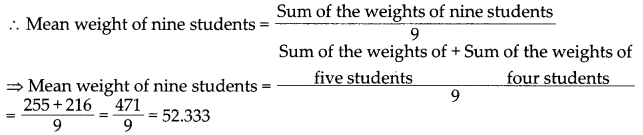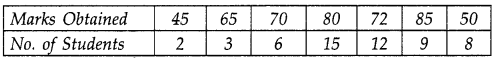# DAV Class 7 Maths Chapter 12 Brain Teasers Solutions

The DAV Maths Class 7 Solutions and DAV Class 7 Maths Chapter 12 Brain Teasers Solutions of Data Handling offer comprehensive answers to textbook questions.

## DAV Class 7 Maths Ch 12 Brain Teasers Solutions

Question 1.
A. Tick (✓) the correct option:
(i) If the mean of 7, 4, x and 10 is 8, the value of x is —
(a) 13
(b) 11
(c) 12
(d) 9
(b) 11

We know that
Mean = $$\frac{\text { Sum of all observations }}{\text { Number of observations }}$$
∴ 8 = $$\frac{7+4+x+10}{4}$$
⇒ 8 = $$\frac{21+x}{4}$$
⇒ 32 = 21 + x
⇒ x = 32 – 21
⇒ x = 11
Hence, (b) is the correct option.

(ii) The observation that occurs maximum number of times in a data is called—
(a) range
(b) median
(c) mode
(d) mean
(c) mode

Mode is the observation which occurs maximum number of times.
Hence, (c) is the correct option.

(iii) The heights(in cm) of six students in a class are 145, 152, 148, 150, 153, 146. The median of the heights is —
(a) 149 cm
(b) 151 cm
(c) 148 cm
(d) 150 cm
(a) 149 cm

Arranging the data in ascending order, we have 145, 146,148,150,152,153 So, two middle most observations are 148 and 150.
∴ Median = $$\frac{\text { Sum of two middle most observations }}{2}$$
= $$\frac{148+150}{2}$$ [Here, median is the average (mean) of two middle most observations (3rd and 4th observation)]
= 149 cm
Hence, (a) is the correct option.

(iv) The mean weight of five students is 51 kg and the mean weight of four students is 54 kg. The mean weight of nine students will be—
(a) less than 52 kg
(b) more than 53 kg
(c) between 52 kg and 53 kg
(d) equal to 51 kg
(c) between 52 kg and 53 kg

Mean weight of five students = 51 kg
∴ Sum of the weights of five students = 5 × 51 kg = 255 kg
Now, mean weight of four students = 54 kg
∴ sum of the weights of four students = 4 × 54 kg = 216 kgHence, (c) is the correct option.

(v) The mean of first ten odd number is
(a) 10
(b) 13
(c) 15
(d) 9
(a) 10

First ten odd numbers are 1, 3, 5, 7, 9, 11, 13, 15, 17, 19
∴ Mean = $$\frac{(1+3+5+7+9+11+13+15+17+19)}{10}=\frac{100}{10}$$ = 10(i) Calculate the mode of 1.6,1.3,1.8, 1.6,1.3,1.1,1.2, 1.3
(ii) Name the three measures of central tendency.
(iii) Find the mean of prime numbers between 70 and 100.
(iv) What is the median of the observations 36, 48, 29, 62, 71 and 84?
(v) The mean of 16 numbers is 8. If 2 is added to every number, find the new mean.
(i) Arranging the data in ascending order, we have
1.1, 1.2, 1.3, 1.3, 1.3, 1.6, 1.6, 1.8
Here, 1.3 occurs most frequently (3 times).
So, the mode is 1.3.

(ii) Mean, Median, Mode are certain representation of a given data. They are called measures of central tendency.

(iii) Prime numbers between 70 and 100 are 71, 73, 79, 83, 89, 97. So, there are six numbers between 70 and 100.
∴ Mean = $$\frac{71+73+79+83+89+97}{6}$$
⇒ Mean = $$\frac{492}{6}$$
⇒ Mean = 82

(iv) Arranging the given observations in ascending order, we have 29, 36, 48, 62, 71, 84
Two middle most observation are 48 and 62.
Median = $$\frac{\text { Sum of two middle most observations }}{2}$$
= $$\frac{48+62}{2}$$ = 55 [Here, median is the average (mean) of two middle most observations (3rd and 4th observation)]

(ii) Mean of 16 numbers = 8
∴ Sum of the 16 numbers = 8 × 16 = 128
If 2 is added to every number, then the sum of the 16 numbers will be [128 + 2(16)]
i. e., 160.
Hence, new mean = $$\frac{160}{16}$$ = 10

Question 2.
Find the mean, median and mode of the given data.
35, 32, 35, 42, 35, 32, 34
Mean: We know that mean = $$\frac{\text { Sum of all observations }}{\text { Number of observations }}$$
Mean = $$\frac{35+32+35+42+35+32+34}{7}=\frac{245}{7}$$
Median: Arranging the data in ascending order, we have 32, 32, 34, 35, 35, 35, 42
We can see that the number of observations is odd, so the median is the middle most observation i.e., 35.
Mode: Arranging the data in ascending form, we have
32, 32, 34, 35, 35, 35, 42
Here, 35 occurs most frequently (3 times).
So, the mode is 35.

Question 3.
The mean of 40 observations was 160. It was detected on rechecking that the value 165 was wrongly copied as 125 for computation of mean. Find the correct mean.
Mean of 40 observations = 160
∴ Sum of the 40 observations = 160 × 40 = 6400
Since, the value 165 was wrongly copied as 125, therefore, the correct sum of all the observations = 6400 + 165 – 125 = 6440
Hence, correct mean = $$\frac{6440}{40}$$ = 161

Question 4.
The mean of 10 numbers is 20. If 5 is subtracted from every number, what will be the new mean?
Mean of 10 numbers = 20
Sum of 10 numbers = 10 × 20 = 200
If 5 is subtracted from every number, then the sum of 10 numbers will be [200 – 5(10)] i.e.’, 150
Hence, new mean = $$\frac{150}{10}$$ = 15

Question 5.
Find the median of the following observations.
46, 64, 87, 41, 58, 77, 35, 90, 55, 92, 33
Arranging the given observations in ascending order, we have
33, 35, 41, 46, 55, 58, 64, 77, 87, 90, 92
We can see that the number of observations is odd, so the median is the middle most observation, i.e., 58.
Now, if 92 is replaced by 99 and 41 by 43 in the given data, then the new observations are:
33, 35, 43, 46, 55, 58, 64, 77, 87, 90, 99
We can see that the number of observations is odd, so the median is the middle most observation, i.e., 58.
Hence, the new median is 58.

Question 6.
Find the mode of 2$$\frac{1}{4}$$, 3.5, 4.1, 3$$\frac{1}{2}$$, 2$$\frac{1}{2}$$, 0.5.
We arrange the given data in the following form:
0. 5, 2$$\frac{1}{4}$$, 2$$\frac{1}{2}$$, 3.5, 3$$\frac{1}{2}$$, 4.1
1. e., 0.5, 2.25, 2.5, 3.5, 3.5, 4.1
Here, we see that 3.5 occurs the most (2 times)
Mode = 3.5Question 7.
The results of pass percentage of Class-X and XII in CBSE examination for five years are given below: –Draw a bar graph to represent the data.
On the horizontal axis, write the years and on the vertical axis, write the pass percentage. Draw bar graphs side by side depicting pass percentage obtained by class-X and XII students.## DAV Class 7 Maths Chapter 12 HOTS

Question 1.
The average age of a class of 35 students is 14 years. If the teacher’s age is included, the average age increases by one year. Find the age of the teacher.
Let the age of the teacher be x years.
Average age of 35 students = 14 years
∴ Sum of all the ages of students = 35 × 14 = 490
Now, according to the question, we have 15 = $$\frac{490+x}{36}$$
⇒ 15 x 36 = 490 + x
⇒ 540 = 490 + x
⇒ x = 540 – 490
⇒ x = 50
So, the age of teacher is 50 years.

Question 2.
The average marks of ten students is 60. If the highest mark is excluded, the average is 59. Find the highest mark.
Let the highest mark be x.
Average marks of 10 students = 60
∴ Sum of all the marks = 10 x 60 = 600
Now, according to the question, we have ^i>59
⇒ 600 – x = 9 × 59
⇒ 600 – x = 531
⇒ x = 600 – 531
⇒ x = 69
So, the highest mark is 69.

Question 3.
The mean of two numbers is 10 and their difference is zero. Find the numbers.
Let the numbers be x and y.
∴ Mean = $$\frac{x+y}{2}$$
10 = $$\frac{x+y}{2}$$
⇒ x + y = 20 …(1)
Also, x – y = 0 (given)
⇒ x = y

Now, Putting the value of x in equation (1), we get
y + y = 20
⇒ 2y = 20
⇒ y = $$\frac{20}{2}$$ = 10
∴ x = y = 10
So, the numbers are 10 and 10.

## DAV Class 7 Maths Chapter 12 Enrichment Questions

Question 1.
Find the mean, median and mode of the following observations.
75%, 3.2, 450%, 0.25, 3$$\frac{1}{5}$$, 1.54.
The given observations are:
75%, 3.2, 450%, 0.25. 3$$\frac{1}{5}$$, 1.54
i.e $$\frac{75}{100}$$, 3.2, $$\frac{450}{100}$$, 0.25, $$\frac{16}{5}$$, 1.54
Now, arranging the given observations in ascending order, we have
0.25, 0.75, 1.54, 3.2, 3.2, 4.5

Mean: Mean = $$\frac{\text { Sum of all observations }}{\text { Number of observations }}$$
∴ Mean = $$\frac{0.25+0.75+1.54+3.2+3.2+4.5}{6}=\frac{13.44}{6}$$ = 2.24

Median: Arranging the data in ascending order, we have
0.25, 0.75, 1.54, 3.2, 3.2, 4.5
We can see that the number of observations is even, so the median is the mean or average of two middle most observations.
∴ Median = $$\frac{\text { Sum of two middle most observations }}{2}=\frac{1.54+3.2}{2}=\frac{4.74}{2}$$ = 2.37

Mode: The given observation in ascending order are:
0.25, 0.75, 1.54, 3.2, 3.2, 4.5
Here, 3.2 occurs most frequently (2 times).
So, the mode is 3.2.

Question 1.
Following are the marks obtained by 10 students in an examination. 65, 70, 75, 80,60, 50, 70, 82, 78, 90
(i) Calculate the mean marks.
(ii) Find the mean marks if a student with 65 marks is also included.
(iii) Find the mean marks if a student having 90 marks is excluded.
(iv) What is the range of marks?
The given marks are;
65, 70, 75, 80, 60, 50, 70, 82, 78, 90
(i) Mean Marks = $$\frac{\text { Sum of all marks }}{\text { Number of students }}$$
= $$\frac{65+70+75+80+60+50+70+82+78+90}{10}=\frac{720}{10}$$ = 72

(ii) If a student having 65 marks is included then the sum of marks of 11 students = 720 + 65 = 785
∴ Mean = $$\frac{785}{11}$$ = 71.36 (approx)

(iii) If a student with marks 90 is excluded then the sum of the marks of remaining 9 students
= 720 – 90 = 630
∴ Mean = $$\frac{630}{9}$$ = 70.

(iv) Range of Marks = 90 – 50 = 40.

Question 2.
The mean of 11 observations is 65. Later on it was observed that a number 75 was misread as 57. Find the correct mean.
Mean of 11 observations = 65
∴ Their sum = 11 × 65 = 715
∴ Difference = 75 – 57 = 18
∴ Correct sum = 715 + 18 = 733 733
∴Correct mean = $$\frac{733}{11}$$ = 66.67 (approx).

Question 3.
Find the mean, median and mode of the following observations.
8, 7, 6, 5, 0, 3, 4, 3, 9, 6, 8, 8, 3, 8, 7.
Mean = $$\frac{\text { Sum of all observations }}{\text { Number of observations }}$$
= $$\frac{8+7+6+5+0+3+4+3+9+6+8+8+3+8+7}{15}=\frac{85}{15}$$ = 5’67 (approx)
Median : Arranging the observations in increasing order,
0, 3, 3, 3, 4, 5, 6, 6, 7, 7, 8, 8, 8, 8, 9
Number of observations = 15
Median = $$\left(\frac{15+1}{2}\right)^{\text {th }}$$ observation
= 8th observation = 6

Mode : In the given observations 8 repeats 4 times.
So, the mode is 8.Question 4.
Find the mode from the following table.